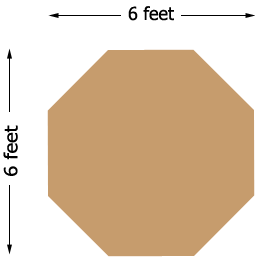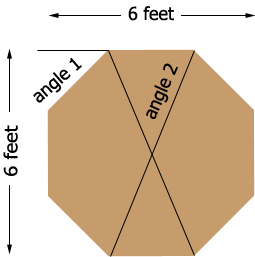SEARCH HOMEMath Central Quandaries & QueriesQuestion from Alan: My grandson and I would like to making a 6'x6' octagon floor for a deer blind. He is eight yrs old and the math is outside my wheelhouse. We need to know the length of the boards and angles of the corners please.Hi Alan,

Is this what you mean by a 6'x6' octagon?If so then you can use the method Stephen used in a question from Martin to show that

the length of a side = 0.4142 times the width,

which in your case give

the length of a side = 0.4142 times 6 feet = 2.485 feet

which is approximately $2 \large \frac12$ feet.

I'm not sure what angle you need. lf you need the exterior angle, angle 1 in my diagram then it's measure is $45^{o}.$If you want the interior angle, angle 2 in my diagram then you can use my response to a question by Trish to show that the measure of this angle is $67.5^{o}.$

Write back if you need more assistance,
PennyMath Central is supported by the University of Regina and The Pacific Institute for the Mathematical Sciences.